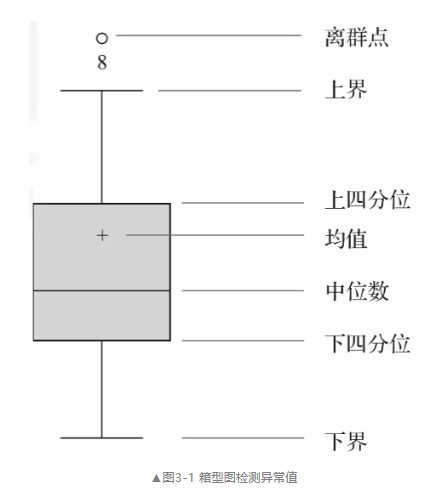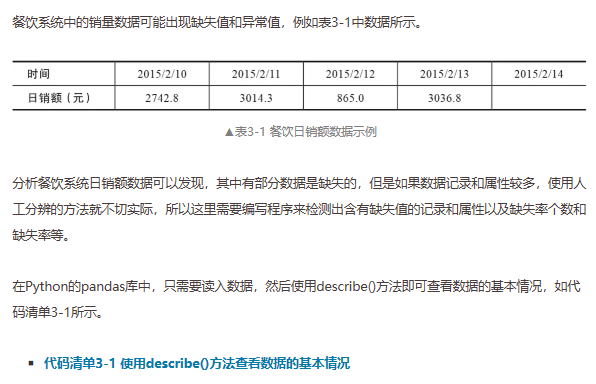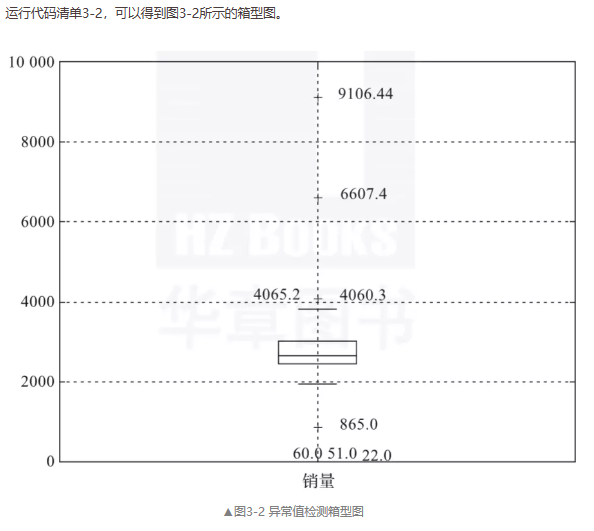#0
0
01. 云栖社区>
2. 云栖号资讯>
3. 博客>
4. 正文

## 什么是脏数据？怎样用箱形图分析异常值？终于有人讲明白了01 缺失值分析

1. 缺失值产生的原因

2. 缺失值的影响

3. 缺失值的分析02 异常值分析

1. 简单统计量分析

1. 3σ原则

1. 箱型图分析

QL称为下四分位数，表示全部观察值中有四分之一的数据取值比它小；
QU称为上四分位数，表示全部观察值中有四分之一的数据取值比它大；
IQR称为四分位数间距，是上四分位数QU与下四分位数QL之差，其间包含了全部观察值的一半。import pandas as pd
catering_sale = '../data/catering_sale.xls'  # 餐饮数据
# 读取数据，指定“日期”列为索引列
print(data.describe())

           销量
count   200.000000
mean   2755.214700
std     751.029772
min      22.000000
25%    2451.975000
50%    2655.850000
75%    3026.125000
max    9106.440000

import matplotlib.pyplot as plt# 导入图像库
plt.rcParams['font.sans-serif'] = ['SimHei']# 用来正常显示中文标签
plt.rcParams['axes.unicode_minus'] = False  # 用来正常显示负号

plt.figure()  # 建立图像
p = data.boxplot(return_type='dict')  # 画箱型图，直接使用DataFrame的方法
x = p['fliers'].get_xdata()  # 'flies'即为异常值的标签
y = p['fliers'].get_ydata()
y.sort()  # 从小到大排序，该方法直接改变原对象
'''

'''
for i in range(len(x)):
if i>0:
plt.annotate(y[i], xy=(x[i],y[i]), xytext=(x[i]+0.05 -0.8/(y[i]-y[i-1]), y[i]))
else:
plt.annotate(y[i], xy=(x[i],y[i]), xytext=(x[i]+0.08,y[i]))

plt.show()  # 展示箱型图03 一致性分析

【云栖号在线课堂】每天都有产品技术专家分享！

【云栖号在线课堂 社群】https://c.tb.cn/F3.Z8gvnK

+ 关注